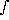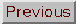### POWER SPECTRUM P(k)

Adapted from P. Coles, 1999, The Routledge Critical Dictionary of the New Cosmology, Routledge Inc., New York. Reprinted with the author's permission. To order this book click here: http://www.routledge-ny.com/books.cfm?isbn=0415923549

While the Universe may be roughly homogeneous and isotropic on the scale of our horizon, as required by the cosmological principle, the distribution of galaxies in space is decidedly inhomogeneous on scales smaller than this (see large-scale structure). In order to quantify the lumpiness of the matter distribution revealed by redshift surveys and to relate this to models of structure formation, cosmologists employ a variety of statistical tools, the most common of which is called the power spectrum and which is usually given the symbol P(k).

The power spectrum is defined in the mathematical language of Fourier series. The simplest way to define it is to define a fluctuation field(x) at different spatial locations x in terms of the actual density of matter(x) at the position x, and subtract the mean density of matter0:(x) = ((x) -0) /0

Because this departure from the mean density is divided by the mean density, the resultingis dimensionless; it is usually called the density contrast.

Suppose we consider a part of the Universe contained within a cubic volume V of side L (V = L3). Assuming (for mathematical purposes only) that the Universe outside this cube consists of periodic replications of what is inside, we can expandin a Fourier series representing a superposition of plane waves with different amplitudes:(x) =(k) exp(ik . x)

The sum is taken over all the waves that fit into the periodic box. These waves have wave-vectors k of the form (kx, ky, kz) = (nx, ny, nz)2/ L, where the nx etc. are integers. The

Fourier coefficients(k) for each mode are complex numbers having both an amplitude and a phase. If instead of the volume V we had chosen a different volume V', we would have found that the same series expansion would be possible but that the co-efficients were a different set of complex numbers. We can imagine repeating this box-shifting idea for a huge number of boxes all around the Universe. It would then be possible to calculate statistical averages over these Fourier coefficients, such as the average squared modulus of(k), which we can write as <(k)* (k) >, where the * denotes a complex conjugate. We can then define the quantityk as<(k)* (k) > = (1 / V)k2

where the summation is now over all modes, and the average is over all possible boxes with the same volume V. We can interpretk as being a kind of average amplitude for waves with wave-vector k. If the distribution of matter is statistically homogeneous and isotropic, then this average will not depend on the direction of k, just its magnitude k. The final step in this definition is now to let the volume V tend to infinity; the right-hand side of the above equation is then

(1 / V)k2 = (1 / 22)P(k)k2 dk

where P(k) is the power spectrum or, more precisely, the power spectral density function. Despite its rather involved derivation, P(k) is quite simple to visualise: it represents the contribution to the fluctuation field from waves of wavenumber k (and therefore wavelengths= 2/k). A completely flat power spectrum corresponds to white noise (i.e. equal power at all frequencies), while a power spectrum that is sharply peaked at some particular value of k has a characteristic length scale. A single plane wave has a power spectrum consisting of a single spike.

In most theories of cosmological structure formation there are initial primordial density fluctuations specified by a very simple power-law form: P(k)kn. In most versions of the inflationary Universe the power spectrum has an index n very close to 1, which is called the scale-invariant or Harrison-Zel'dovich spectrum. The situation is a little more complicated than this in realistic models of structure formation, because this initial spectral shape can be modified by non-gravitational processes in the period before recombination: Silk damping, free streaming and the Meszaros effect can all alter the shape of P(k). Given any particular detailed model, however, these effects can be modelled and they are usually represented in terms of a transfer function T(k) for the model such that

Output P(k) = Input P(k) x T(k)2

Knowing T(k) then allows us to define the initial conditions for a detailed computation of the evolution of density fluctuations.

The advantages of the power spectrum as a way of characterising density fields are many. Firstly, in the gravitational Jeans instability, as long as the fluctuations are small (much less than 1) we can solve the equations describing their evolution using perturbation theory. We find that the shape of the power spectrum is not changed by gravitational evolution: only the amplitude increases as a function of time. To put this another way, in perturbation theory all the Fourier modes evolve independently, so that the amplitude of each mode increases at the same rate. Assuming that the power spectrum decreases with decreasing k (increasing wavelength), which it must do if the Universe is to be smooth on large scales, this means that the power spectrum for small k should retain its primordial shape. This allows astronomers to probe the primordial density fluctuations directly by using observations of galaxy clustering.

On smaller scales where nonlinear (non-perturbative) effects are important, the power spectrum changes shape but it does not lose its usefulness. We can compute the power spectrum in an N-body simulation of a model, for example, and use it to test the model in question against observations, from which the power spectrum can be estimated quite easily.

Another advantage for the initial stages of clustering evolution is linked to the statistical properties of the initial fluctuations. In most theories, including the inflationary Universe models, the initial seed irregularities are Gaussian. This means that the power spectrum alone furnishes a complete statistical description of the fluctuations. Know the power spectrum, and you know it all.

There is also an important connection between P(k) and the method that has been historically important for quantifying the distribution of galaxies in space: the two-point correlation function,(r). Together these two functions form a Fourier transform pair, so they provide completely equivalent information about the fluctuation field. A very similar concept to P(k) can be used to describe the fluctuations in temperature on the (two-dimensional) celestial sphere, rather than in three-dimensional space.

FURTHER READING:

Harrison, E.R., `Fluctuations at the threshold of classical cosmology', Physical Review D, 1970, 1, 2726. Zel'dovich, Ya.B., `A hypothesis unifying the structure and entropy of the Universe', Monthly Notices of the Royal Astronomical Society, 1972, 160, 1P. Coles, P., `The large-scale structure of the Universe', Contemporary Physics, 1996, 37, 429. Coles, R and Lucchin, F., Cosmology: The Origin and Evolution of Cosmic Structure (John Wiley, Chichester, 1995), Chapter 14.